# How to Fill Same Texts/Comments in Filtered List in Excel

Sometimes we want to add the same texts or comments for filtered values in a filtered list. Normally we can add texts or comments into the first cell and then drag the fill handle down to fill the other filtered cells with the same texts. But if you cannot apply this due to some limits in some special situations, you can follow below method to add the same texts or comments in filtered cells. Please read article below, and let’s started to learn the method.

Precondition: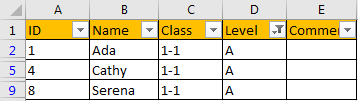We want to add the same comment ‘Pass’ for all filtered students in Comment column.

## Method: How to Fill Same Texts/Comments in Filtered List

Step 1: Select E2 to E4, then press F5 key to trigger Go To dialog.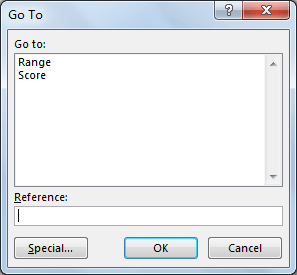Step 2: Click Special button in the bottom. Verify that Go To Special dialog pops up.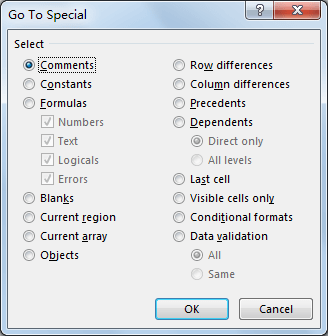Step 3: Check on Visible cells only option. Then click OK.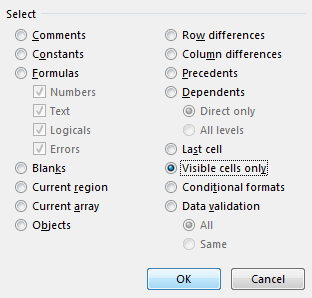Step 4: After above step, you can notice that only visible cells E2 to E4 are selected. Press Space on keyboard, then the first cell (E2 in this case) is in edit mode. Type ‘Pass’ into E2.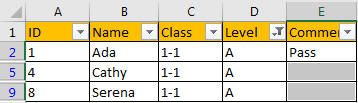Step 5: Press Ctrl+Enter simultaneously. Verify that the other two filtered cells are also filled with ‘Pass’ properly. And the hidden cells will not be filled with comments. You can also apply this method to update cells.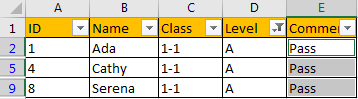Related Posts

Cap percentage values between 0 and 100

This article will talk about how to cap the percentage values between 0% and 100% in Microsoft Excel Spreadsheet or Google Sheets. If you are a newbie on Excel or google Sheets, you may be able to do this by ...

Calculate Cap Percentages to Specific Value

This article will talk about how to limit the cap percentage of a given amount to a given value in Microsoft Excel Spreadsheet or Google Sheets. If you are a newbie on Excel or google Sheets, you may be able ...

Calculate Win Loss Tie

Suppose you got a task to calculate the win, loss, and tie totals; what would you do? If you are new to Ms Excel and don't have enough experience with it, then you might do this task manually but let ...

Calculate Years Between Dates In Ms Excel

If you are an avid Ms Excel user, then you might have come across a task in which you needed to calculate the years between the dates; you might take it easy and do this task manually, which is also ...

Calculate Number of Hours between Two Times

Calculating the difference between two times might be a valuable statistic for subsequent computations or averages, whether you're producing a time sheet for staff or recording personal exercises. While Excel has a plethora of complex functions, including date and time ...

Calculate Loan Interest in Given Year

When you borrow money, you are supposed to repay it gradually. Lenders, on the other hand, want to be compensated for their services and the risk they incur by lending you money. That is, you will not just repay the ...

Calculate Interest Rate for Loan

The interest rate is the fee charged by a lender to a borrower and is expressed as a percentage of the principal—the lent amount. The interest rate on a loan is often expressed as an annual percentage rate, abbreviated as ...

Calculate Interest for Given Period

Using the IPMT function in Excel, we can compute the interest payment on any loan. This step-by-step tutorial will guide Excel users of all skill levels through the process to calculate interest for given period. Finally, the formula: =IPMT(B3/12,1,B5,-B2) The ...

How To Use Excel GCD Function

This post will guide you how to use Excel GCD function with syntax and examples in Microsoft excel. Description The Excel GCD function Returns the greatest common divisor of two or more integers. So you can use the GCD function ...

Calculate A Ratio From Two Numbers In Excel

In elementary mathematics, a ratio is a connection or comparison between two or more integers. For example, ratios are often expressed as ":" to demonstrate the relationship between two numbers. You would think that manually calculating a ratio from two ...

Sidebar